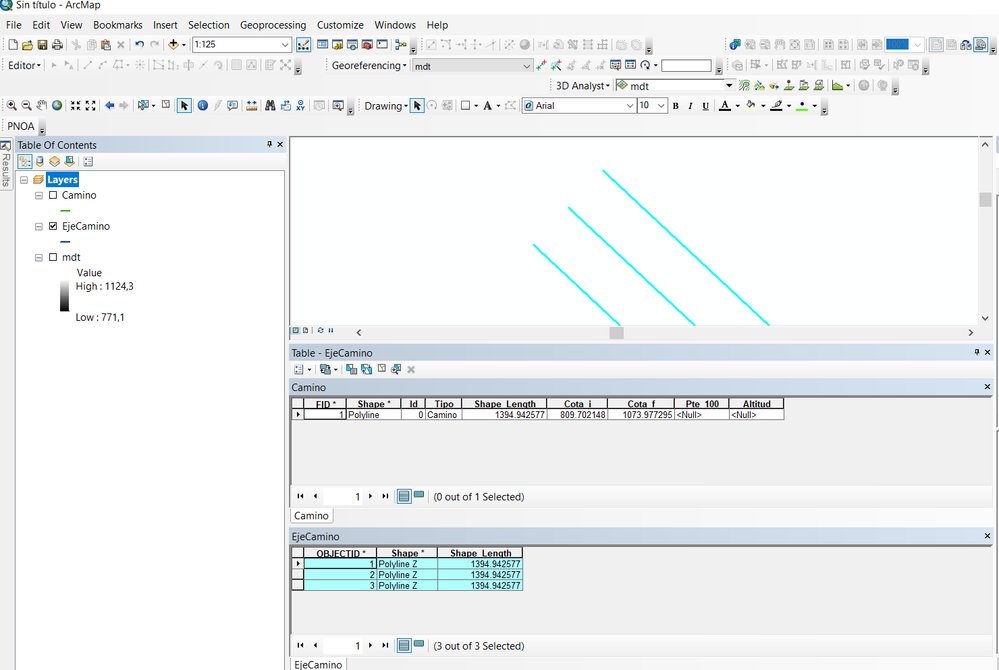# Fill Z vertex in Polyline 3d

520
4
02-16-2023 08:29 AMNew Contributor II

My goal is to modify the Z coordinates of a 3d polyline (Camino3d)  from the fields of an attribute table of another feature (Camino).``````layer = r'C:\Desmonte_terraplen_vs01.gdb\Camino'
fc_dest = r"C:\Desmonte_terraplen_vs01.gdb\Camino_3D"
with arcpy.da.SearchCursor(layer,["Cota_i","Cota_f"]) as search_cur:
for search_row in search_cur:
with arcpy.da.UpdateCursor(fc_dest,["SHAPE@Z"], explode_to_points=True) as upd_cur:
for upd_row in upd_cur:
upd_row = search_row
upd_cur.updateRow(upd_row)``````

Using Explode to point = True I split the polyline into multipoints and I think I can iterate over them.
The problem comes in that I don't know how to iterate inside the Z values of the 3d polyline.
Any suggestions? Thanks a lot!

Tags (1)
1 Solution

Accepted SolutionsbyMVP Frequent Contributor

The problem comes in that I don't know how to iterate inside the Z values of the 3d polyline.

A polyline is basically a twodimensional array of vertices:

polyline = [  [point, point, point],  [point, point, point, point, point]  ]

The geometry and its parts are iterable objects, the vertices are arcpy.Point objects.

``````shape = [r for r in arcpy.da.SearchCursor(layer, ["SHAPE@"])]
print("Shape: " + str(shape))
for part in shape:
print("\tPart: " + str(part))
for vertex in part:
print("\t\tVertex: " + str(vertex))
print("\t\t\tCoordinates: " + str([vertex.X, vertex.Y, vertex.Z, vertex.M]))``````

You have to construct a new line geometry, updating the vertices as points probably won't get you anywhere.

Assuming that layer and dest_fc are in the same order:

``````# read the elevation values
endpoint_elevations = [row
for row in arcpy.da.SearchCursor(layer, ["Cota_i", "Cota_f"])
]

# start the update cursor for the complete shape token, not just z
with arcpy.da.UpdateCursor(fc_dest, ["SHAPE@"]) as cursor:
for i, row in enumerate(cursor):
# get the corresponding elevation values
start, end = endpoint_elevations[i]
# interpolate the elevation for each vertex
# if your fc is m-aware, you can also uncomment the two lines to update the m values
shape = row
part = shape
new_part = []
slope = (end - start) / shape.length
m_value = 0
for v, vertex in enumerate(part):
if v == 0:
vertex.Z = start
else:
v0 = part[v-1]
v1 = vertex
m_value += ((v1.X - v0.X)**2 + (v1.Y - v0.Y)**2)**0.5
vertex.Z = start + slope * m_value
#vertex.M = m_value
new_part.append(vertex)
# create a new shape and update the feature
new_shape = arcpy.Polyline(arcpy.Array(new_part),
spatial_reference=shape.spatialReference,
#has_m=True,
has_z=True
)
cursor.updateRow([new_shape])
``````

Have a great day!
Johannes
4 RepliesbyMVP Frequent Contributor

The problem comes in that I don't know how to iterate inside the Z values of the 3d polyline.

A polyline is basically a twodimensional array of vertices:

polyline = [  [point, point, point],  [point, point, point, point, point]  ]

The geometry and its parts are iterable objects, the vertices are arcpy.Point objects.

``````shape = [r for r in arcpy.da.SearchCursor(layer, ["SHAPE@"])]
print("Shape: " + str(shape))
for part in shape:
print("\tPart: " + str(part))
for vertex in part:
print("\t\tVertex: " + str(vertex))
print("\t\t\tCoordinates: " + str([vertex.X, vertex.Y, vertex.Z, vertex.M]))``````

You have to construct a new line geometry, updating the vertices as points probably won't get you anywhere.

Assuming that layer and dest_fc are in the same order:

``````# read the elevation values
endpoint_elevations = [row
for row in arcpy.da.SearchCursor(layer, ["Cota_i", "Cota_f"])
]

# start the update cursor for the complete shape token, not just z
with arcpy.da.UpdateCursor(fc_dest, ["SHAPE@"]) as cursor:
for i, row in enumerate(cursor):
# get the corresponding elevation values
start, end = endpoint_elevations[i]
# interpolate the elevation for each vertex
# if your fc is m-aware, you can also uncomment the two lines to update the m values
shape = row
part = shape
new_part = []
slope = (end - start) / shape.length
m_value = 0
for v, vertex in enumerate(part):
if v == 0:
vertex.Z = start
else:
v0 = part[v-1]
v1 = vertex
m_value += ((v1.X - v0.X)**2 + (v1.Y - v0.Y)**2)**0.5
vertex.Z = start + slope * m_value
#vertex.M = m_value
new_part.append(vertex)
# create a new shape and update the feature
new_shape = arcpy.Polyline(arcpy.Array(new_part),
spatial_reference=shape.spatialReference,
#has_m=True,
has_z=True
)
cursor.updateRow([new_shape])
``````

Have a great day!
JohannesNew Contributor II

Great response!
I have modified the parameters of the polyline creation tool and it works great.
If I wanted to loop through a feature with more entities to do the same thing, how should I do the loop?byMVP Frequent Contributor

If the features in Camino and Camino_3D are in the same order, you can just use this script.

If your screenshot was just for testing and you have another structure, you need to explain that structure.

Have a great day!
JohannesNew Contributor II

If you mean geometry type and attribute, Camino and Camino3d are the same structure.
What happens is that for what I am doing, I have defined the axis of the path (Camino3d) and a right and left margin (three 3d polylines inside the same feature with the same Z dimension) inside the EjeCamino feature (sorry for the strange names)